• 学生考试信息管理功能主要包括两大功能模块，学生操作模块和教师操作模块。其中学生操作模块分为两个部分，一是账号管理功能（其中包含登录账号、密码修改以及找回密码等），二是信息查询功能。在信息查询部分，学生...
• 系统利用Java技术和MYSQL技术，成功实现了考试管理系统常备的学生信息的增、删、改、查以及老师基本信息的修改；基本上具备了系统完备的功能。
• 学生考试管理系统源码学生考试管理系统源码学生考试管理系统源码
• 学生考试管理系统课程设计报告，用户对应相应的权限进行登录，分教员、学生、管理员。登录后根据选择的权限进入相应的界面。有代码
• ## 学生选课管理信息系统

万次阅读 多人点赞 2019-01-04 11:55:23
学生入校注册后需统一记录学生个人基本信息，对于面向学生开设的相关课程需要记录每门课程的基本信息，每个任课教师规定其可主讲三门课程，学生选课时系统将相应的选课信息记录入库，考试结束后需在相应的选课记录中...
文件下载地址：https://download.csdn.net/download/axiebuzhen/10895062

1．业务描述

设计本系统，模拟学生选课的部分管理功能。学生入校注册后需统一记录学生个人基本信息，对于面向学生开设的相关课程需要记录每门课程的基本信息，每个任课教师规定其可主讲三门课程，学生选课时系统将相应的选课信息记录入库，考试结束后需在相应的选课记录中补上考试成绩。简化的系统数据库（SCDB）中主要包含以下五个数据表（设计时可根据实际业务需要适当追加其它的数据表），各表及其相应字段分别描述如下：

（1）学生信息表（student）

学号sno：char(9)

姓名sname：nvarchar(8)

性别ssex：nchar(1)    男　或　女

年龄sage：int(4)     14≤sage≤24

系别sdept：nvarchar(30)

（2）课程信息表（course）

课程号cno：char(6)

课程名cname：nvarchar(50)

先行课编号cpno：char(6)

学分ccredit：smallint(2)

（3）教师信息表（teacher）

教工号tno：char(8)

姓名tname：nvarchar(8)

性别tsex：nchar(1)    男　或　女

年龄tage：int(4)     24≤sage≤60

学历teb：nvarchar(10)    学士、硕士、博士

职称tpt：nvarchar(10)    助教、讲师、副教授、教授

主讲课程一cno1: char(6)

主讲课程二cno2: char(6)

主讲课程三cno3: char(6)

（4）院系信息表（department）

系编号dno：char(3)

系名dname：varchar(30)

系主任dmanager：char(8)

（5）选课信息表（sct）

学号sno：char(9)

课程号cno：char(6)

教工号tno：char(8)

不多说，运行界面：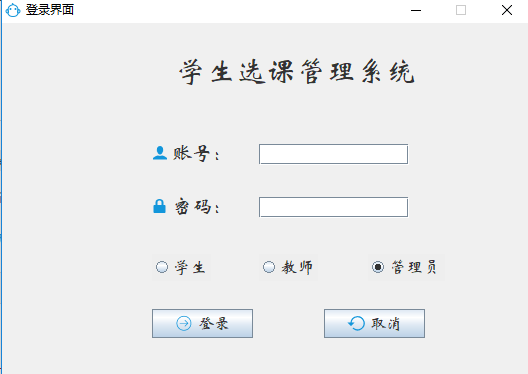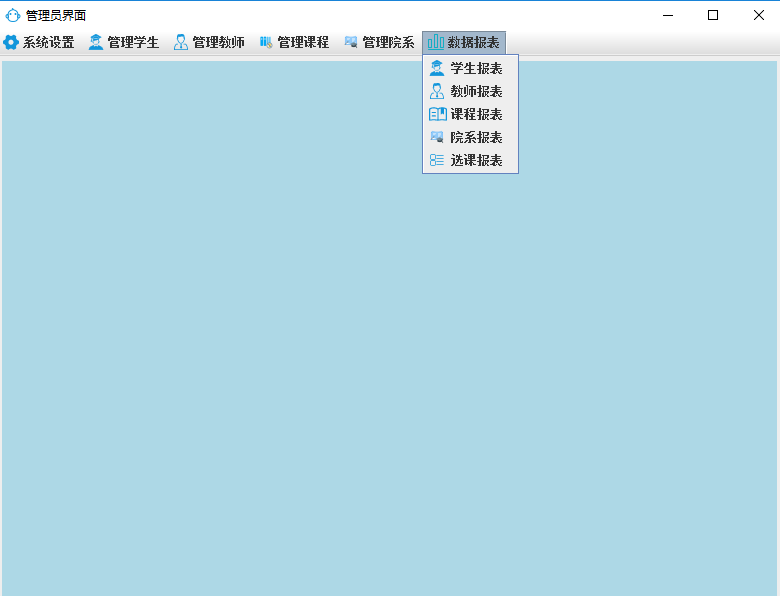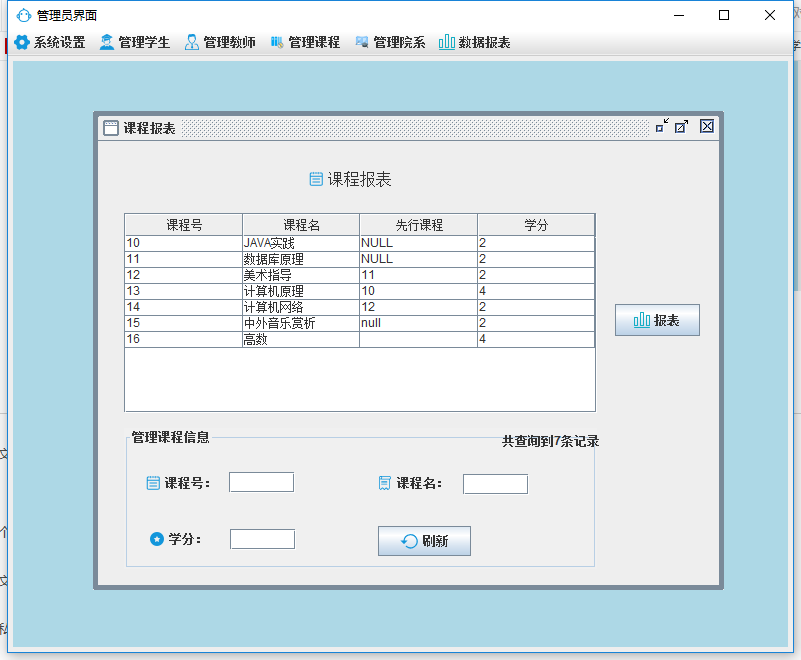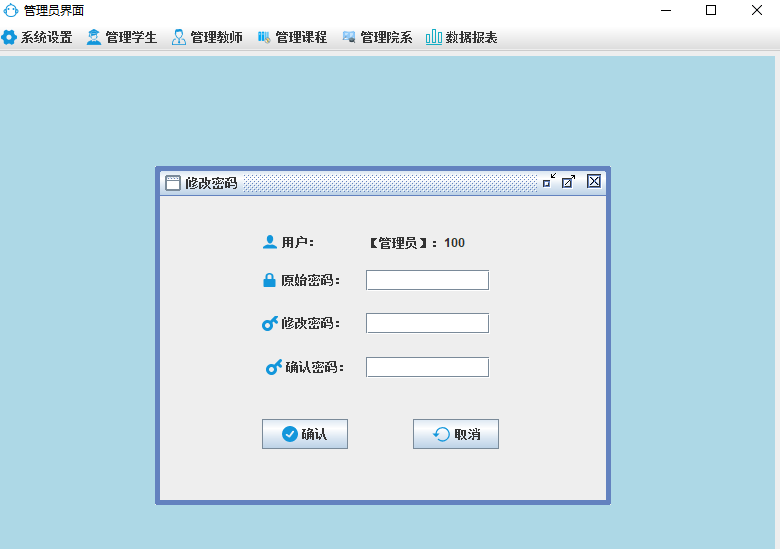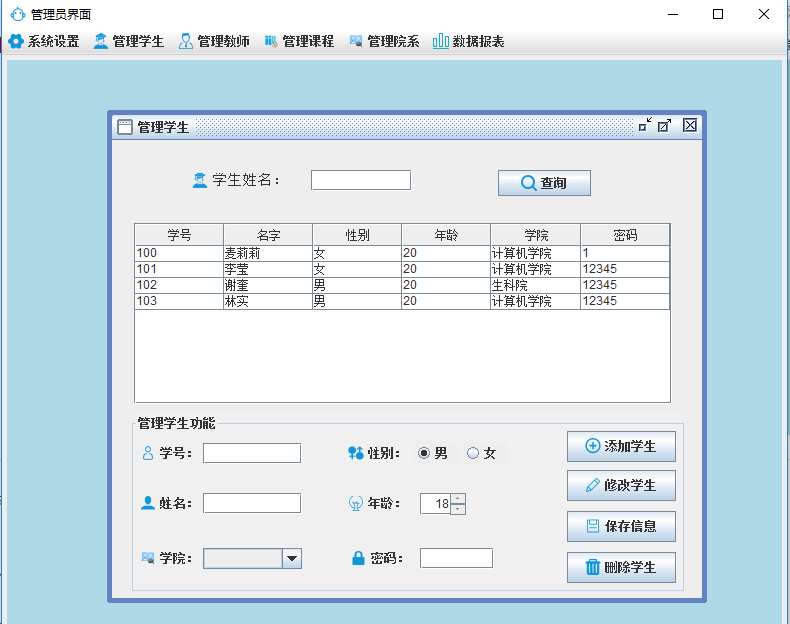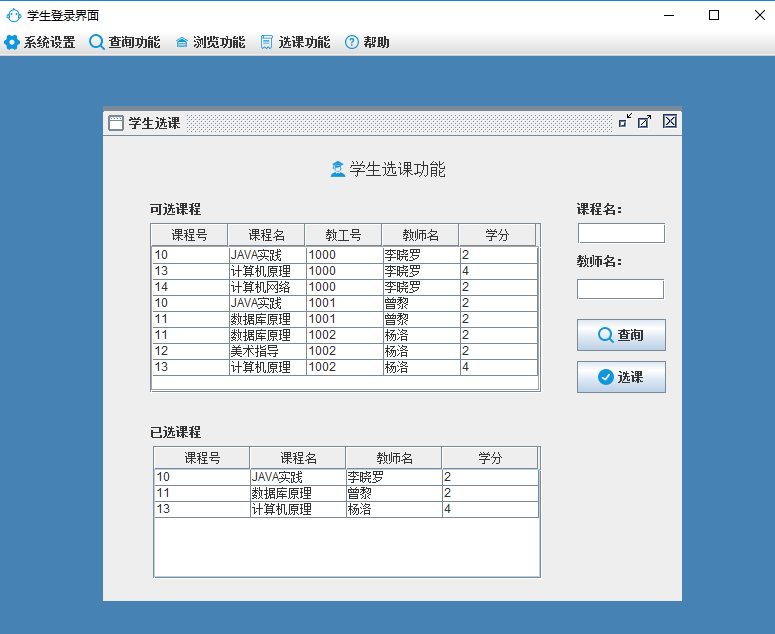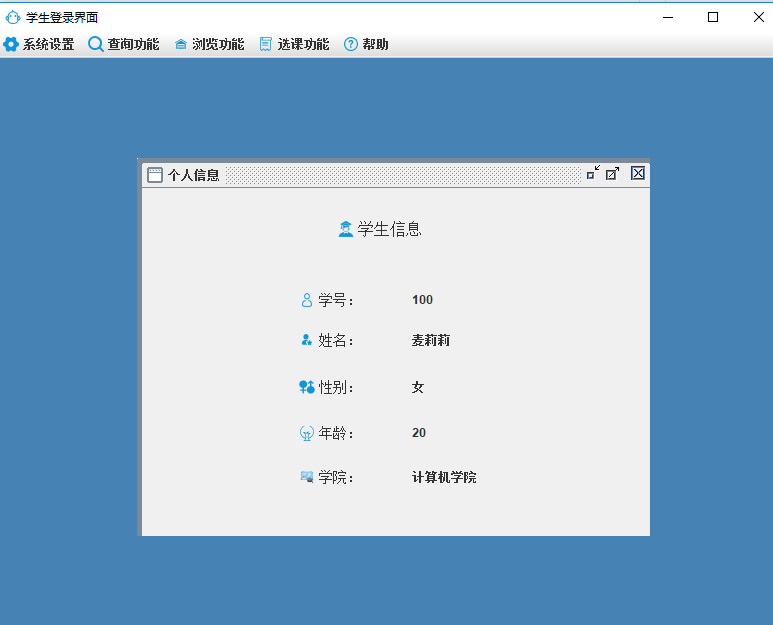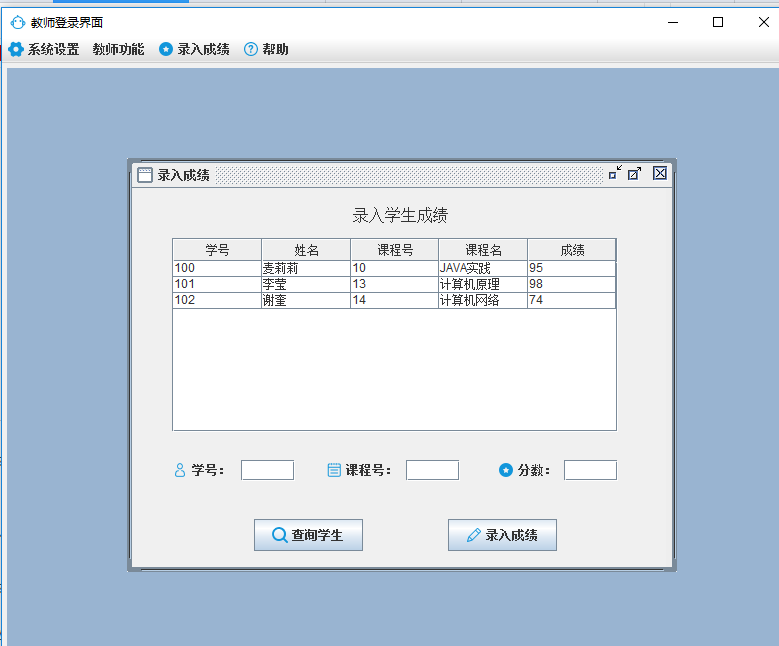展开全文• 学生管理信息系统；图书馆信息服务系统；教务处课程安排系统；企业物流管理系统；企业通讯管理系统；网络考试综合系统；人力资源管理系统；。。。完整源程序+以上各系统PDF电子书(工作安排系统除外)! 相当实用的资料...
• 本系统是基于C/S模式的采用vb实现的学生管理信息系统（Students Management Information System），以后简称SMIS。数据库服务器由access实现。操作系统：WINDOWS xp；数据库服务器端软件Microsoft Access2000 + SQL...
• ## 简单学生成绩管理信息系统

万次阅读 多人点赞 2018-06-14 21:01:56
简单学生成绩管理信息系统 学生成绩信息包括：学号，姓名，课程名，平时成绩，实验成绩，考试成绩，总评成绩。实现如下功能：1) 能够实现学生成绩信息的插入、删除和修改；2) 能够实现各种查询（分别根据学生学号...
简单学生成绩管理信息系统 学生成绩信息包括：学号，姓名，课程名，平时成绩，实验成绩，考试成绩，总评成绩。实现如下功能：1)      能够实现学生成绩信息的插入、删除和修改；2)      能够实现各种查询（分别根据学生学号、姓名、课程名称等）；3)      能够实现按照考试成绩、总评成绩进行排序；4)      能够查询某门课程的最高分、最低分并输出相应学生信息；5)      能够查询某门课程的优秀率（90 分及以上）、不及格率；#include <stdio.h>#include <stdlib.h>#include <string.h>struct student{	int number;	char name;	char banji; 	char course;	int pscore;	int kscore;	int sumsore;	struct student *next;  } ; struct student *create()    //先构造一个结构体 {	struct student *head;   	struct student *p1;		p1=(struct student*)malloc(sizeof(struct student));  //申请一个空间	head=p1;		printf("\n学生信息\n");    //输入学生的信息 	printf("学号:");	scanf("%d",&p1->number);	printf("姓名:");	scanf("%s",p1->name);	printf("班级：");	scanf("%s",p1->banji);	printf("课程名:");	scanf("%s",p1->course);		printf("平时成绩:");	scanf("%d",&p1->pscore);		printf("考试成绩:");	scanf("%d",&p1->kscore);	printf("总评成绩:");	scanf("%d",&p1->sumsore);			return head;		} struct student *input(int n)  //输入学生信息 {   int i;   char y ;   struct student *head,*pt,*p;   head=pt;   p=(struct student*)malloc(sizeof(struct student));  //申请一个空间      if(i!=1)  //判断是不是第一空间    {     for(i=1;i<n;i++)     {       	 pt=pt->next;     }      pt->next=create();    }    else    {    	pt=create();	 }       pt=pt->next;      pt->next=p;   pt=pt->next;   pt->next=NULL;        return head;}void amend(struct student *head)   //修改学生信息 {	int m,k,fale=0;	char z;	struct student *p; 	  do	   {			p=head;			printf("请输入要修改的学生的学号：");	scanf("%d",&m);		do	{	  if(p->number==m)  //寻找该学号的学生 	  {	  	printf("学号:%d\n姓名:%s\n班级：%s\n课程名:%s\n平时成绩:%d\n考试成绩:%d\n总评成绩:%d\n",p->number,p->name,p->banji,p->course,p->pscore,p->kscore,p->sumsore);	  	printf("\n1.学号\n2.姓名\n3.班级\n4.课程名\n5.平时成绩\n6.考试成绩\n7.总评成绩\n0.不修改");	  	printf("\n请输入要修改：");		scanf("%d",&k);		  		printf("修改为：");		  		 switch(k)  //对学生学号进行修改 		 {		 	case 1:scanf("%d",&p->number);break;		 	case 2:scanf("%s",p->name);break;		 	case 3:scanf("%s",p->banji);break; 		    case 4:scanf("%s",p->course);break;		    case 5:scanf("%d",&p->pscore);break;		    case 6:scanf("%d",&p->kscore);break;		    case 7:scanf("%d",&p->sumsore);break;		    case 0:break;		  };		   		 fale=1;		 break;	  }			p=p->next;				}while(p->next!=NULL);		if(fale==0)   printf("没有查找到这个人。\n");    	 	printf("是否继续修改（Y/N）:");  //是否还进行修改的判断 	getchar();	scanf ("%c",&z);	  }while(z!='n'&&z!='N');	}void camcel(struct student *head)   // 删除学生信息{	int i=1,m,k,fale=0;	char z;	struct student *p,*pt; 	  do	   {			p=head;	pt=head;	p=p->next; 			printf("请输入要删除的学生的学号：");	scanf("%d",&m);		do	{	  if(p->number==m)   //寻找该学生 	  {	  	printf("学号:%d\n姓名:%s\n班级：%s\n课程名:%s\n平时成绩:%d\n考试成绩:%d\n总评成绩:%d\n",p->number,p->name,p->banji,p->course,p->pscore,p->kscore,p->sumsore);	  	printf("\n是否要删除（Y/N）:");   	  	getchar();		scanf("%c",&k);		  		if(k=='y'||k=='Y')   //对学生删除的操作 		{		  if(i==1)    //先判断是否是第一个学生 		  {		  	head->next=p->next;		  }		   else		   {             do             {        	 pt=pt->next;          		     }while(pt->next!=p);		     		     pt->next=p->next;		     free(p);           }	    }		 fale=1;		 break;	  }		i++;		p=p->next;				}while(p->next!=NULL);		if(fale==0)   printf("没有查找到这个人。\n"); 		printf("是否继续修改（Y/N）:");	getchar();	scanf ("%c",&z);	  }while(z!='n'&&z!='N');	}void xingmingchaxun(struct student *head) //按姓名查询 {	struct student *p;	char a,c;	int fale=0;	  do   {   	p=head;	printf("输入要查找的姓名：");	scanf("%s",a);		do	{	   if(strcmp(p->name,a)==0)			{	  	printf("学号:%d  姓名:%s   班级：%s  课程名:%s  平时成绩:%d  考试成绩:%d  总评成绩:%d  \n",p->number,p->name,p->banji,p->course,p->pscore,p->kscore,p->sumsore);	  	fale=1;	  	c=1;		}				p=p->next;			}while(p!=NULL);		if(fale==0) printf("\n没有查找到该学生\n");		printf("\n\n是否继续查找（Y/N）:");	getchar();	scanf ("%c",&c);	    }while(c!='n'&&c!='N');		} void kechengchaxun(struct student *head)// 按课程名称查询{	struct student *p;	char a,c;	int fale=0;	  do   {   	p=head;   	printf("\n输入要查找的课程：");	scanf("%s",a);   		do	{	   if(strcmp(p->course,a)==0)			{	  	printf("学号:%d  姓名:%s   班级：%s  课程名:%s  平时成绩:%d  考试成绩:%d  总评成绩:%d  \n",p->number,p->name,p->banji,p->course,p->pscore,p->kscore,p->sumsore);	  	fale=1;	  	c=1;		}				p=p->next;			}while(p!=NULL);		if(fale==0) printf("\n没有查找到该课程\n");		printf("\n\n是否继续查找（Y/N）:");	getchar();	scanf ("%c",&c);	    }while(c!='n'&&c!='N');		} void xuehaochaxun(struct student *head) // 按学号查询{	struct student *p;	char c;	int a,fale=0;	  do   {   	p=head;   	printf("\n输入要查找的学号：");	scanf("%d",&a);   		do	{	   if(p->number==a)			{	  	printf("学号:%d  姓名:%s   班级：%s  课程名:%s  平时成绩:%d  考试成绩:%d  总评成绩:%d \n ",p->number,p->name,p->banji,p->course,p->pscore,p->kscore,p->sumsore);	  	fale=1;	  	c=1;		}				p=p->next;			}while(p!=NULL);		if(fale==0) printf("\n没有查找到该学号的学生\n");		printf("\n\n是否继续查找（Y/N）:");	getchar();	scanf ("%c",&c);	    }while(c!='n'&&c!='N');} void banjichaxun(struct student *head)  //按班级查询 {	struct student *p;	char a,c;	int fale=0;	  do   {   	p=head;   	printf("\n输入要查找的班级：");	scanf("%s",a);   		do	{	   if(strcmp(p->banji,a)==0)			{	  	printf("学号:%d  姓名:%s   班级：%s  课程名:%s  平时成绩:%d  考试成绩:%d  总评成绩:%d  ",p->number,p->name,p->banji,p->course,p->pscore,p->kscore,p->sumsore);	  	fale=1;	  	c=1;		}				p=p->next;			}while(p!=NULL);		if(fale==0) printf("\n没有查找到该班级\n");		printf("\n\n是否继续查找（Y/N）:");	getchar();	scanf ("%c",&c);	    }while(c!='n'&&c!='N');} void youxiulv(struct student *head)  //查询班级优秀率 {	struct student *p;	char a,c;	float n=0,m=-2;	  do   {   	p=head;   	printf("\n输入要查找的优秀率的科目：");	scanf("%s",a);   		do	{	   if(strcmp(p->course,a)==0)			{          if(p->sumsore>=80)		        n++;		}				m++;				p=p->next;			}while(p!=NULL);	    printf("该目的优秀率为：%0.2f",n/m) ;		printf("\n\n是否继续查找（Y/N）:");	getchar();	scanf ("%c",&c);	    }while(c!='n'&&c!='N');} void zongfengpaixu(struct student *head,int n)  //按总分高低排序 {	char c;	int i,j;	struct student *p,temp;	struct student a[n];			p=head;	p=p->next;		for(i=0;i<=n-1;i++)	{		a[i].number=p->number;		strcpy(a[i].name,p->name);		strcpy(a[i].banji,p->banji);		strcpy(a[i].course,p->course);		a[i].pscore=p->pscore;		a[i].kscore=p->kscore;		a[i].sumsore=p->sumsore; 		p=p->next;	}		for(i=0;i<=n-1;i++)    //冒泡法来排列顺序      {  	   for(j=0;j<=n-2;j++)  	  {  		if(a[j].sumsore<a[j+1].sumsore)  		{  			temp=a[j];  			a[j]=a[j+1];  			a[j+1]=temp;		  }      			  }    }        printf("Nes:\n");	do	{ 	      for(i=0;i<n;i++)     {     	printf("学号:%d  姓名:%s   班级：%s  课程名:%s  平时成绩:%d  考试成绩:%d  总评成绩:%d  \n",a[i].number,a[i].name,a[i].banji,a[i].course,a[i].pscore,a[i].kscore,a[i].sumsore);	 }	 	printf("\n\n是否退出（Y/N）:");	getchar();	scanf ("%c",&c);	    }while(c!='y'&&c!='Y');   } void dankechengjipaiming(struct student *head,int n)  //单科成绩排名 {	char c,b;	int i,j,m;	struct student *p,temp;	struct student a[n];		do	{	m=0;	p=head;	p=p->next;		printf("输入要查找的科目：");	scanf("%s",b);        for(i=0;i<=n-1;i++)       	{	  if(strcmp(p->course,b)==0)	   {		a[m].number=p->number;		strcpy(a[m].name,p->name);		strcpy(a[m].banji,p->banji);		strcpy(a[m].course,p->course);		a[m].pscore=p->pscore;		a[m].kscore=p->kscore;		a[m].sumsore=p->sumsore; 				 m++;	   }		p=p->next;    }     	for(i=0;i<=m-1;i++)     //冒泡法来排列顺序      {  	   for(j=0;j<=m-2;j++)  	  {  		if(a[j].sumsore<a[j+1].sumsore)  		{  			temp=a[j];  			a[j]=a[j+1];  			a[j+1]=temp;		  }      			  }    }    	printf("Nes:\n");	      for(i=0;i<m;i++)     {     	printf("学号:%d  姓名:%s   班级：%s  课程名:%s  平时成绩:%d  考试成绩:%d  总评成绩:%d  \n",a[i].number,a[i].name,a[i].banji,a[i].course,a[i].pscore,a[i].kscore,a[i].sumsore);	 }	 	printf("\n\n是否退出（Y/N）:");	getchar();	scanf ("%c",&c);	    }while(c!='y'&&c!='Y');	}void pingshifengpaiming(struct student *head,int n){	char c;	int i,j;	struct student *p,temp;	struct student a[n];			p=head;	p=p->next;		for(i=0;i<=n-1;i++)	{		a[i].number=p->number;		strcpy(a[i].name,p->name);		strcpy(a[i].banji,p->banji);		strcpy(a[i].course,p->course);		a[i].pscore=p->pscore;		a[i].kscore=p->kscore;		a[i].sumsore=p->sumsore; 		p=p->next;	}		for(i=0;i<=n-1;i++)    //冒泡法来排列顺序      {  	   for(j=0;j<=n-2;j++)  	  {  		if(a[j].pscore<a[j+1].pscore)  		{  			temp=a[j];  			a[j]=a[j+1];  			a[j+1]=temp;		  }      			  }    }        printf("Nes:\n");	do	{ 	      for(i=0;i<n;i++)     {     	printf("学号:%d  姓名:%s   班级：%s  课程名:%s  平时成绩:%d  考试成绩:%d  总评成绩:%d  \n",a[i].number,a[i].name,a[i].banji,a[i].course,a[i].pscore,a[i].kscore,a[i].sumsore);	 }	 	printf("\n\n是否退出（Y/N）:");	getchar();	scanf ("%c",&c);	    }while(c!='y'&&c!='Y');}void kaoshifengpaiming(struct student *head,int n){	char c;	int i,j;	struct student *p,temp;	struct student a[n];			p=head;	p=p->next;		for(i=0;i<=n-1;i++)	{		a[i].number=p->number;		strcpy(a[i].name,p->name);		strcpy(a[i].banji,p->banji);		strcpy(a[i].course,p->course);		a[i].pscore=p->pscore;		a[i].kscore=p->kscore;		a[i].sumsore=p->sumsore; 		p=p->next;	}		for(i=0;i<=n-1;i++)    //冒泡法来排列顺序      {  	   for(j=0;j<=n-2;j++)  	  {  		if(a[j].kscore<a[j+1].kscore)  		{  			temp=a[j];  			a[j]=a[j+1];  			a[j+1]=temp;		  }      			  }    }        printf("Nes:\n");	do	{ 	      for(i=0;i<n;i++)     {     	printf("学号:%d  姓名:%s   班级：%s  课程名:%s  平时成绩:%d  考试成绩:%d  总评成绩:%d  \n",a[i].number,a[i].name,a[i].banji,a[i].course,a[i].pscore,a[i].kscore,a[i].sumsore);	 }	 	printf("\n\n是否退出（Y/N）:");	getchar();	scanf ("%c",&c);	    }while(c!='y'&&c!='Y');	}void pingshichengjichaxun(struct student *head,int n){	struct student *p;	char c;	int a,fale=0;	  do   {   	p=head;   	printf("\n输入要查找的平时成绩：");	scanf("%d",a);   		do	{	   if(a==p->pscore)			{	  	printf("学号:%d  姓名:%s   班级：%s  课程名:%s  平时成绩:%d  考试成绩:%d  总评成绩:%d  \n",p->number,p->name,p->banji,p->course,p->pscore,p->kscore,p->sumsore);	  	fale=1;	  	c=1;		}				p=p->next;			}while(p!=NULL);		if(fale==0) printf("\n没有查找到该成绩\n");		printf("\n\n是否继续查找（Y/N）:");	getchar();	scanf ("%c",&c);	    }while(c!='n'&&c!='N');}void kaoshichengjichaxun(struct student *head,int n){		struct student *p;	char c;	int a,fale=0;	  do   {   	p=head;   	printf("\n输入要查找的考试成绩：");	scanf("%d",a);   		do	{	   if(a==p->kscore)			{	  	printf("学号:%d  姓名:%s   班级：%s  课程名:%s  平时成绩:%d  考试成绩:%d  总评成绩:%d  \n",p->number,p->name,p->banji,p->course,p->pscore,p->kscore,p->sumsore);	  	fale=1;	  	c=1;		}				p=p->next;			}while(p!=NULL);		if(fale==0) printf("\n没有查找到该成绩\n");		printf("\n\n是否继续查找（Y/N）:");	getchar();	scanf ("%c",&c);	    }while(c!='n'&&c!='N');}int jisuan(char a[]){	int i,sum=0;		for(i=0;a[i]!='\0';i++)	{		if(i==0)		{			sum=a[i]-'0';		}		else		{			sum=sum*10+(a[i]-'0');		}			}return sum;}int main(){	int n=0,ch=0,th=0;	int *q;	q=&n;		struct student *intput_shuji(int *m);	struct student *create() ;	struct student *input(int n);	void amend(struct student *head) ;	void camcel(struct student *head);	void xingmingchaxun(struct student *head);	void kechengchaxun(struct student *head);	void xuehaochaxun(struct student *head);	void banjichaxun(struct student *head);	void youxiulv(struct student *head);	void zongfengpaixu(struct student *head,int n);	void dankechengjipaiming(struct student *head,int n);	void pingshifengpaiming(struct student *head,int n);	void kaoshifengpaiming(struct student *head,int n);	void pingshichengjichaxun(struct student *head,int n);	void kaoshichengjichaxun(struct student *head,int n);		struct student *head;	do	{		printf("学生成绩管理系统\n\n");    printf("1.学生数据输入\n2.学生数据修改\n3.学生数据查找\n4.学生排名\n5.退出系统") ;	printf("\n----------------------------------------\n") ;		printf("\n要进行的步骤");		scanf("%d",&ch) ;	 system("cls");				 switch(ch)	 {	 	case 1:{				n++;	 		    head=input(n);				break;				}					    	    case 2:{	    	   do	 		   {	 		   	printf("\n1.修改学生信息\n2.删除学生信息\n3.返回上一层\n");	 		   	printf("\n要进行的步骤:");	 		   	scanf("%d",&th);	 		   		 		   	switch(th)	 		   	{	 		   		case 1: amend(head);;break;					case 2:{							camcel(head);	    	                n--;			                break;					       }		         }		          system("cls");		    	}while(th!=3);		    	break;		       }		    case 3:{	    	   do	 		   {	 		   	printf("\n1.按姓名查询\n2.按班级查询\n3.按学号查询\n4.按课程名称查询\n5.按平时成绩查询\n6.按考试成绩查询\n7.按总成绩查询\n8.返回上一层\n");	 		   	printf("\n要进行的步骤:");	 		   	scanf("%d",&th);	 		   		 		   	switch(th)	 		   	{	 		   		case 1:xingmingchaxun(head);break;					case 2:banjichaxun(head); break;		            case 3:xuehaochaxun(head);break;		            case 4:kechengchaxun(head);break;		            case 5:pingshichengjichaxun(head,n);break;		            case 6:kaoshichengjichaxun(head,n);break;		            case 7:zongfengpaixu(head,n);break;				}				system("cls");		    	}while(th!=8);		    	break;		       }		    case 4:{		       do	 		   {	 		   	printf("\n1.按总分高低排序\n2.单科成绩排名\n3.平时分排名\n4.考试分排名\n5.查询班级优秀率\n6.返回上一层\n");	 		   	printf("\n要进行的步骤:");	 		   	scanf("%d",&th);	 		   		 		   	switch(th)	 		   	{	 		   		case 1:zongfengpaixu(head,n);break;					case 2: dankechengjipaiming(head,n);break;		            case 3:pingshifengpaiming(head,n);break;		            case 4:kaoshifengpaiming(head,n);break;		            case 5: youxiulv(head); break;				}				 system("cls");		    	}while(th!=6);               }                               break;            }     system("cls");         }while(ch!=5) ;    	return 0; }
展开全文• vc++简易学生考试管理系统，分两大功能，学生成绩管理和学生信息管理，功能不太多，完成基本的学生信息录入、查询、管理，可以先看一下 测试抓图，比较标准的C++窗体应用，就当作是一个VC操作数据库的参考实例 吧。...
源码下载简介

vc++简易学生考试管理系统，分两大功能，学生成绩管理和学生信息管理，功能不太多，完成基本的学生信息录入、查询、管理，可以先看一下 测试抓图，比较标准的C++窗体应用，就当作是一个VC操作数据库的参考实例 吧。

源码下载地址：点击下载

备用下载地址：点击下载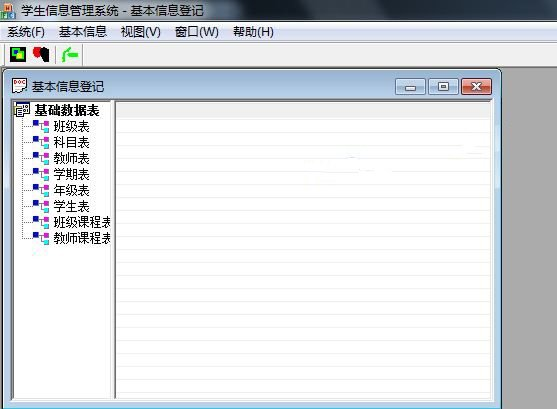展开全文数据库 源代码
• 系统是一个学生管理系统，主要应用于大学的学生管理。由于对系统的分析可能不够全面，只能是模拟一下学生管理系统。每个学生都有一个学号，且没有相同的，所以可以以学号为帐号，这样，同学们就可以自行登陆学生...
• 基于PHP的学生在线考试管理系统分为前后台，可部署在常规集成环境下 (XAMPP WAMP phpstudy) 用户分为学生和管理员 系统使用技术**（laravel+css+javascript）** 二 主要功能 1.管理员制定试卷【单选，多选，判断】和...
一 项目介绍
基于PHP的学生在线考试管理系统分为前后台，可部署在常规集成环境下
(XAMPP WAMP phpstudy)
用户分为学生和管理员
系统使用技术**（laravel+css+javascript）**
二 主要功能
1.管理员制定试卷【单选，多选，判断】和试卷信息的增删改
2.管理员添加修改学生信息
3.学生测试
4.题库随机抽取
5.登陆退出
三 系统界面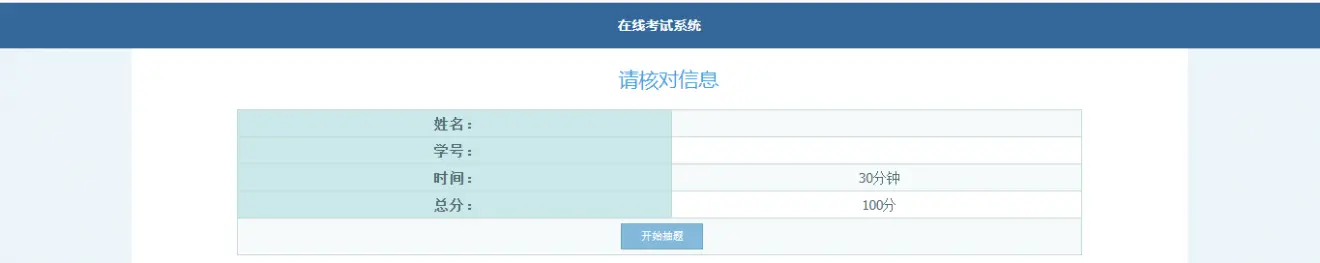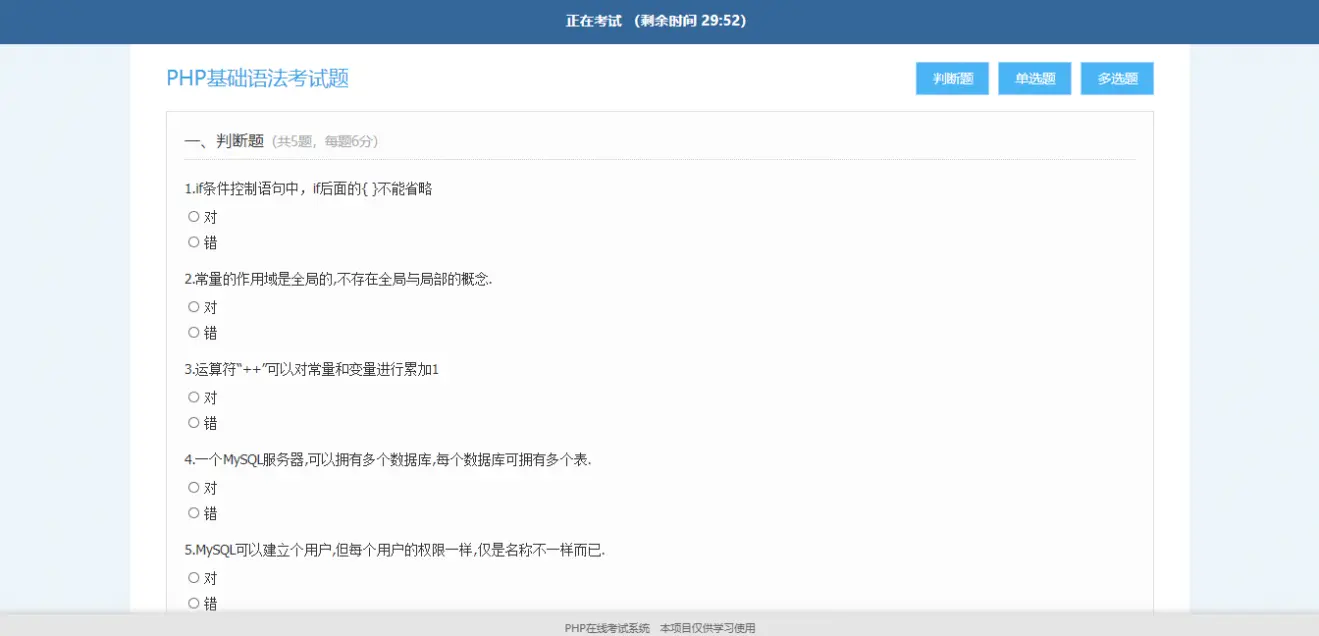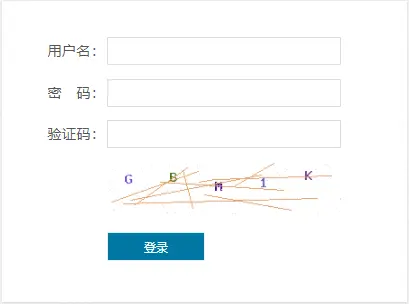四 系统源码与部署可以搭建在phpstudy下，3分钟快速部署
1 数据库配置文件
common/db.ini
2 数据库文件
main/mytest.sql
3 系统管理员
五 FAQ
项目包部署！
hello world！


展开全文php
• margin-top: 10px"> 按姓名查询"> 查询"> 增加学生信息" onclick="window.location.href='stuAddForm.jsp'"> </form> <table border="1" cellspacing="0" align="center"> <tr> 学号</th> 姓名</th> 性别</th> 生日...
• 练习一：请画出学生信息管理系统的用例图 “学生信息管理系统” 功能性需求包括以下内容：  （1）系统管理员登录后可以对班级的基本信息进行增加、删除、修改、查询等操作。学校领导登录后可以对班级基本信息进行...Uml
• 文章目录数据库设计（结构化设计方法）——学生考试管理系统作业要求一、需求分析1.1、需求说明1.2、数据流图1.2.1、系统的顶层数据流图1.2.2、系统的第0层数据流图1.3、数据字典1.3.1、数据流1.3.2、数据存储1.3.3...数据库
• 建议现在还是大一大二的学生们，可以在年级低的时候准备软考中级，这样就算高级的没考过，也有一证在手。并且把软考的相关知识打好基础。这样为以后的考试可以节约很多时间。 对于项管，之前也是了解不多。现在谈谈...任务
• ## C语言学生成绩管理系统源代码

万次阅读 多人点赞 2018-03-21 20:27:20
大学C语言实训课，C语言学生成绩管理系统。 #include<stdio.h> #include<string.h> #include<math.h> struct student { int num; char name; float pingshi; float shiyan; ...
• 项目编号：BS-XX-067 运行环境： ... 本项目基于Springboot开发实现一套在线考试及试题管理系统。基本上完成了在线考试系统所需要的相应基本功能。系统基本功能完整，有部分小功能未实现，基本上完...java毕业设计
• （2）管理员可以在系统中进行添加试题、查询学生信息、添加考试学生信息地。（3）学生用户通过自己的考号、姓名登陆可以登录考试系统参加考试和进行成绩的查询。 网上考试系统以方便、满足客户需要为宗旨，实现阅卷...
• 输入学生信息：输入信息包括学生姓名，性别，学生ID,以及学生考试的两门课的成绩。 显示学生信息：显示用户所输入所有的学生信息。 查询学生信息：查询已入录的信息，要求有按姓名查找和按ID查找两种方式。 更新学生...
• 第1章 信息系统项目管理考试论文写作要求 论文涉及的类别 项目绩效考核与绩效管理 团队绩效与项目绩效的关系 绩效评估方法 项目绩效指标设计 绩效改进 大型、复杂信息系统项目和多项目的管理考试的重中之...笔记
• ## 学生信息管理系统

千次阅读 2017-06-20 12:48:00数据库
• ## 学生信息管理系统——总结

千次阅读 热门讨论 2013-08-10 21:06:12
学生信息管理系统是针对学校人事处的大量业务处理工作而开发的管理软件，主要用于学校学生信息管理，总体任务是实现学生信息关系的系统化、科学化、规范化和自动化，其主要任务是用计算机对学生各种信息进行日常管理...数据库
• python实现学生信息管理系统 my_dict={} #先定义一个空字典用来存放数据 #操作页面 def show_menu(): print("---------------------------") print(" 学生管理系统 V1.0") print(" 1:添加学生") ...python
• 和我徒弟一样发文纪念下，信息系统项目管理考试45分，我报好名，开始复习，具体时间，自己去某网站看，上面写着倒计时70天，也不知道对不对。把我 一次通过信息系统项目管理考试过程进行一个简单回顾, 供各位考...
• 学生信息管理系统 表: 学生信息表 入学的时候 --学号[主关键字] 姓名 性别 班级 籍贯 考试成绩表 考试的时候生产的 --学号[] 姓名 成绩 班级 教师表 老师 --名字 工号 教龄 班级 功能: 学生信息...sqlite3数据库 C语言
• 题目学生信息管理系统学生综合评测系统 学生信息管理系统 学生综合评测系统Python
• 考试成绩管理系统 由于篇幅原因，对于系统的UML建模，我们仅给出系统的需求分析和各种建模元素的图例。本附录介绍的是一个考试成绩管理系统。 1.1需求分析 考试成绩管理系统是举行成人高考、自学考试等成人高校对每...
• ## 考试报名管理系统

千次阅读 2017-12-21 09:49:30
/***********************************************************...*内容摘要：该代码用于创建一个考试报名管理系统 *其它说明：无 *当前版本：V1.0 *作者：刘思源 *完成日期：20171221 **修改记录1： *修改日期：20171数据结构 c语言
• 个人通讯录管理系统 要求： 1、用结构体数组存储通信录； 2、每项通信录至少有姓名、电话和备注三条信息； 3、要有友好的交互界面； 4、通信录通过文件存放在当前目前下“通信录.txt”，方便文件的读写； 5、利用......